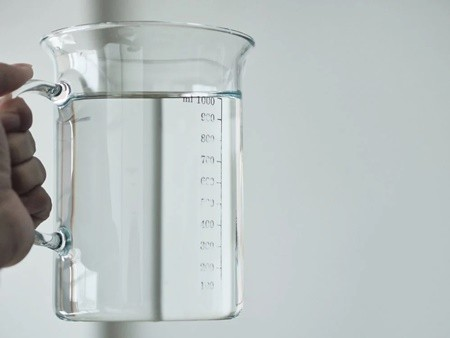# How Many Ounces in a Quart? A Complete Guide to Volume Conversions

Understanding liquid measurements is an essential kitchen skill, whether you’re following recipes or simply need to know how much water fits in a container. A common question is: how many ounces are in a quart? Read on for a full guide to quarts, ounces, and converting between volume units.## Introduction to Quarts and Ounces

First, let’s review what quarts and ounces measure:

• quart is a unit of volume equal to 32 fluid ounces. It’s commonly used to measure liquids in cooking and recipes.
• An ounce can refer to either a unit of weight or volume. A fluid ounce measures the volume or capacity of liquids.

Since we want to know how many fluid ounces fit in a liquid quart, it’s important to note we are working with fluid ounces, not ounces of weight.

## Volume Measurement Systems

The quart and fluid ounce are part of the US customary system of measurement used in the United States. But other places use metric or imperial measurements.

### United States Customary

The US customary system uses quarts, gallons, fluid ounces, cups, pints, and more to measure liquid volume.

### Imperial System

Used in the UK, imperial units like the imperial pint and gallon are slightly larger than US customary units.

### Metric System

The metric system uses milliliters (mL) and liters (L) to measure volume. 1 liter = 1000 mL.

## Converting Ounces to Quarts

Now we can get to the conversion:

### Conversion Formula

There are 32 fluid ounces (fl oz) in 1 quart. To convert ounces to quarts:

Quarts = Ounces / 32

Divide the ounces by 32 to get the equivalent quarts.

For example, 64 fl oz / 32 = 2 quarts

### Quick Conversion

As a handy guide:

• 16 fl oz = 0.5 quart
• 32 fl oz = 1 quart
• 64 fl oz = 2 quarts

And so on, since there are 32 fl oz per quart.

### Conversion Table

Refer to this table when converting ounces to quarts:

Fluid Ounces Quarts
8 fl oz 0.25 qt
16 fl oz 0.5 qt
24 fl oz 0.75 qt
32 fl oz 1 qt
40 fl oz 1.25 qt

Use the formula for conversions not listed.

## Converting Quarts to Other Units

We can also convert quarts to cups, pints, gallons, and liters:

### Cups

1 quart = 4 cups

To get cups, multiply quarts by 4.

### Pints

1 quart = 2 pints

Multiply quarts by 2 to convert to pints.

### Gallons

1 gallon = 4 quarts

Divide quarts by 4 to get gallons.

### Liters

1 quart = 0.946 liters

Multiply quarts by 0.946 to convert to liters.

## Accurate Measurements for Cooking

Converting between units helps ensure accuracy:

### Weighing Ingredients

For precision, weigh ingredients like flour and sugar instead of using cup measurements, which can vary.

### Using Units Consistently

Follow recipe units consistently. Don’t substitute cup for ounces measurements.

### U.S. Customary Units

U.S. recipes typically use cups, tablespoons, and fluid ounces.

### Metric Units

Metric recipes use milliliters, liters, and grams instead of quarts and ounces.

## Conversion Tools

To make conversions easier, use:

• Online converters and calculators
• Apps like Volume for Android and iOS
• Conversion charts

These tools provide instant quart-to-ounce conversions and vice versa.

### How many cups are in 1 quart?

There are 4 cups in 1 quart, since 1 quart = 32 fl oz and 1 cup = 8 fl oz.

### How many fluid ounces in 1 liter?

There are approximately 33.8 fluid ounces in 1 liter. To convert liters to fluid ounces, multiply liters by 33.8.

### What’s the difference between a fluid ounce and a regular ounce?

A fluid ounce measures volume, while a regular ounce measures weight. There are 16 regular ounces in 1 pound.

1 quart is approximately 0.946 liters. To convert quarts to liters, multiply quarts by 0.946.

### Should I use fluid ounces or milliliters in recipes?

Use the units specified in each recipe to ensure accuracy. Don’t substitute one for the other.

## Conclusion

• There are 32 fluid ounces in 1 US liquid quart.
• Dividing total ounces by 32 gives you the equivalent quarts.
• Quarts can also be converted to cups, pints, gallons, and liters using simple multipliers.
• Online tools make fluid ounce and quart conversions easy.

Knowing quart and fluid ounce equivalents allows for accurate, reliable measurements in recipes and cooking.Welcome to MenuPricesLists.com! My name is John, and I am the founder and chief editor of this website. With over 10 years of experience in the food industry, I have dedicated myself to providing accurate, up-to-date, and comprehensive information on menu prices for a wide range of restaurants.